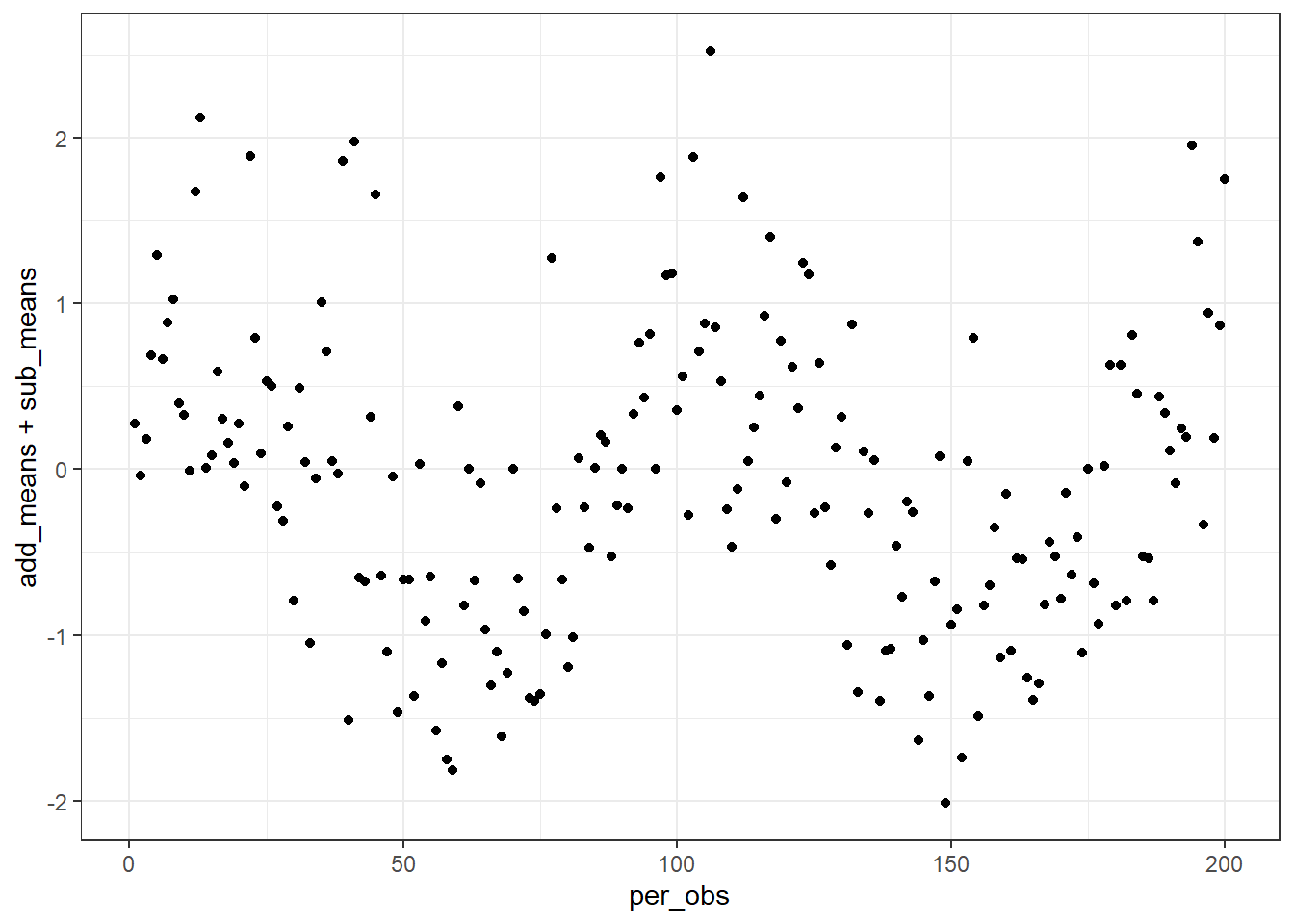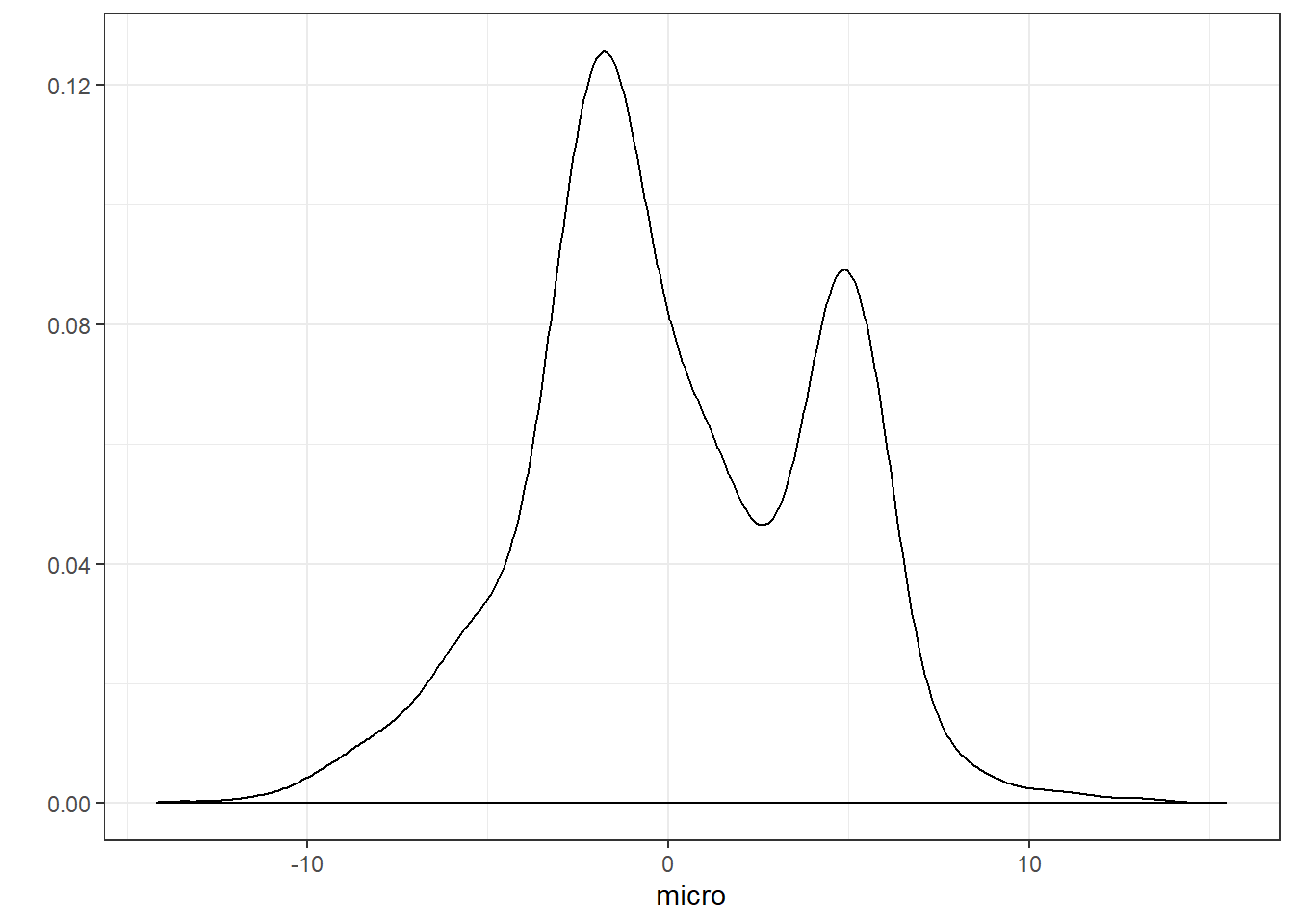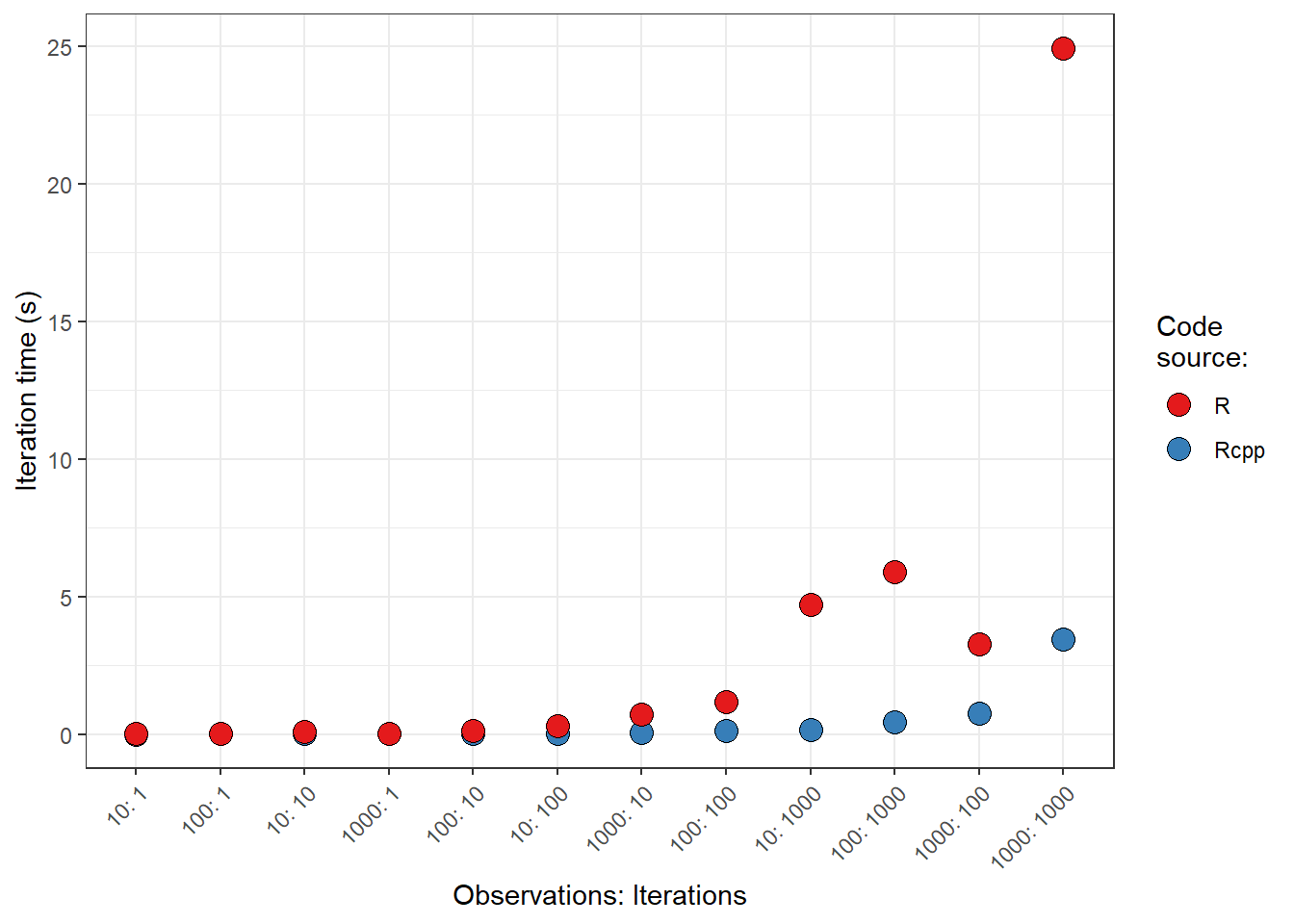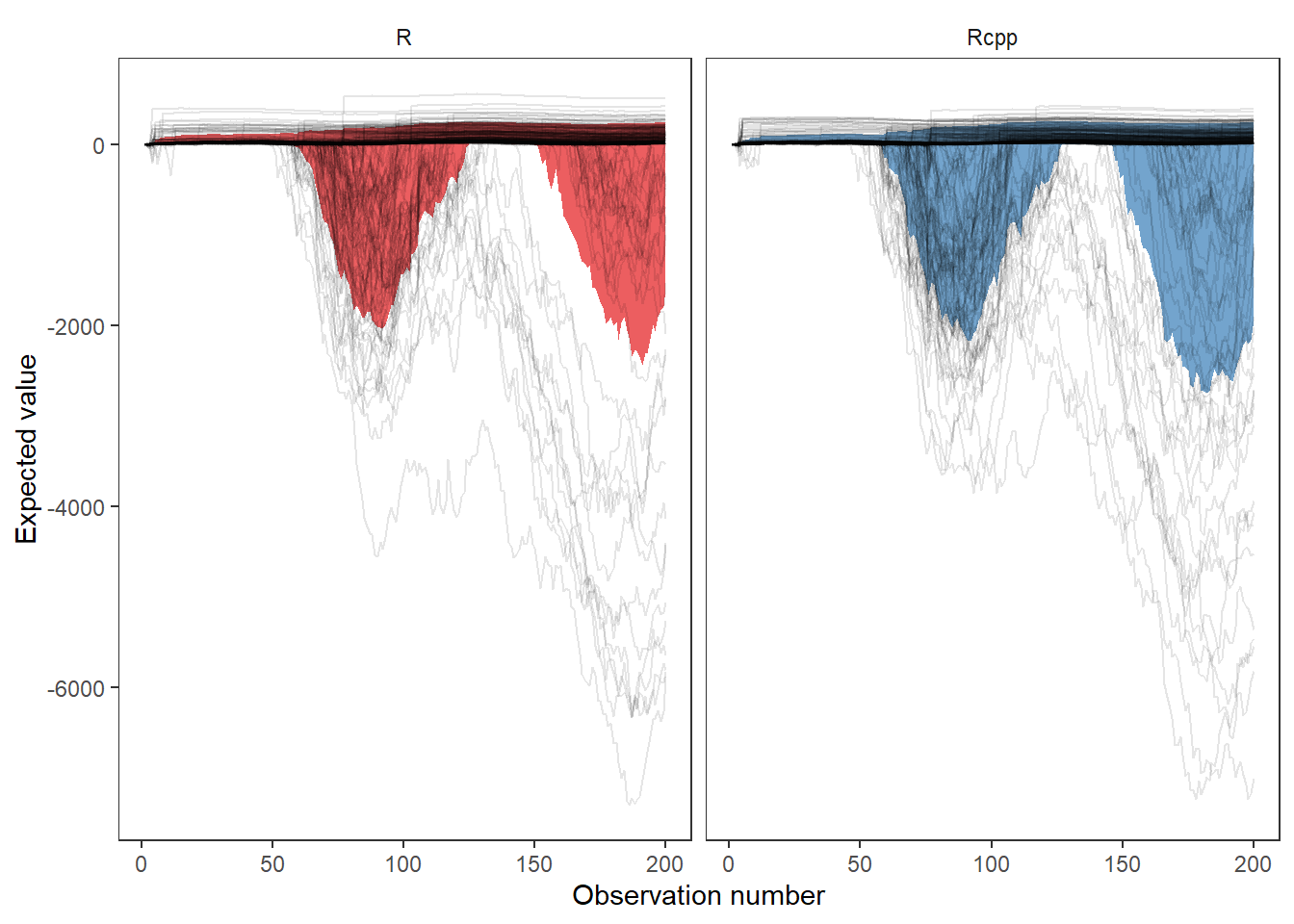# Benchmarking R and Rcpp

The intent of this document is to illustrate some of the efficiencies that can be gained from implementing functions in Rcpp compared to their higher level implementations in R.

## Setup

knitr::opts_chunk$set( echo = TRUE, out.width = "80%" ) library(tidyverse) library(Rcpp) options(tibble.width = Inf) ## R equations The set of functions being used to illustrate differences between R code and Rcpp code. The main function being test is: full_balance. sy <- function(x, cond) { out <- ifelse(cond < x, 1, 0) out <- 1 - (sum(out) / length(x)) ifelse(out <= 0.01, 0.01, out) } new_cond <- function(curr, addit, subtrac, microt) { sy_cond <- sy(microt, curr) curr + (addit + subtrac) / sy_cond } full_balance <- function(iterations, initial, addition, subtraction, add_sd, sub_sd, microtopo) { #Initialize N <- length(addition) #rows M <- iterations #columns out <- matrix(nrow = N, ncol = M) out[1,] <- initial add_error <- matrix(rep(addition, times = M) + rnorm(N * M, 0, add_sd), nrow = N, ncol = M, byrow = FALSE) sub_error <- matrix(rep(subtraction, times = M) + rnorm(N * M, 0, sub_sd), nrow = N, ncol = M, byrow = FALSE) #Execute for(i in 1:iterations) { #columns for(j in 2:length(addition)) { #rows out[j, i] <- new_cond(out[j - 1, i], add_error[j, i], sub_error[j, i], microtopo) } } #Return as.data.frame(out) } ## C++ (Rcpp) equations Similar functions to those above, but implemented in C++ via the Rcpp package. The main function being test is: full_balance_cpp. #include <Rcpp.h> using namespace Rcpp; // [[Rcpp::export]] double sy_cpp(NumericVector x, double cond) { //Declare int N = x.length(); NumericVector cond_sum(N); double out; //First ifelse() // Short-hand form: for(int i = 0; i < N; i++) { cond_sum[i] = (cond < x[i] ? 1 : 0); } //out line out = 1 - (sum(cond_sum) / N); //Second ifelse() if(out <= 0.01) { out = 0.01; } //Explicit return statement return out; } // [[Rcpp::export]] double new_cond_cpp(double curr, double addit, double subtrac, NumericVector microt) { //Declare double sy_cond; double out; //Execute sy_cond = sy_cpp(microt, curr); out = curr + (addit + subtrac) / sy_cond; //Return return out; } // [[Rcpp::export]] DataFrame full_balance_cpp(int iterations, double initial, NumericVector addition, NumericVector subtraction, double add_sd, double sub_sd, NumericVector microtopo) { //Declare int N = addition.length(); //Rows int M = iterations; //Columns NumericMatrix add_error(N, M); NumericMatrix sub_error(N, M); NumericMatrix out_mat(N, M); //Initialize //Set initial conditions for each iteration for(int i = 0; i < M; ++i) { out_mat(0,i) = initial; //Note out() instead of out[] for indexing matrices } //Execute //Matrix of observations with error for(int i = 0; i < M; ++i) { add_error(_, i) = addition + rnorm(N, 0 , add_sd); sub_error(_, i) = subtraction + rnorm(N, 0 , add_sd); } //Loop through each set of observations for(int i = 0; i < M; ++i) { for(int j = 1; j < N; ++j){ out_mat(j, i) = new_cond_cpp(out_mat(j - 1, i), add_error(j, i), sub_error(j, i), microtopo); } } //Return a dataframe of results //Explicit coercion to a different, compatable type following the pattern: // as<type>(object) DataFrame out = as<DataFrame>(out_mat); return out; } ## Data Some data being used test the different functions. per_obs <- 1:200 per_n <- length(per_obs) add_means <- 0.5 * (sin(seq(0, 2 * per_n / 100 * pi, length.out = per_n))) + abs(rnorm(per_obs)) add_means <- ifelse(add_means < 0 , 0, add_means) sub_means <- 0.75 * (cos(seq(0, 2 * per_n / 100 * pi, length.out = per_n)) - 2) + abs(rnorm(per_obs)) sub_means <- ifelse(sub_means > 0 , 0, sub_means) add_sds <- 1 sub_sds <- 1 init_cond <- 0 micro <- map2(.x = c(-4, -2, 0, 2, 5), .y = c(3, 1, 2, 4, 1), ~ rnorm(2000, .x, .y)) %>% simplify() ## Data visualization Just to give an idea of what the data looks like. This problem was originally conceived of as a time series. qplot(per_obs, add_means + sub_means) + theme_bw()qplot(micro, geom = "density") + theme_bw()## Benchmarking Here I’m using the bench package to iterate over different parameter sets, so we may get an idea of how the two equivalent functions respond to changes in function conditions. ### Run the benchmarks micro_maker <- function(obs) { map2(.x = c(-4, -2, 0, 2, 5), .y = c(3, 1, 2, 4, 1), ~ rnorm(obs, .x, .y)) %>% simplify() } iter_maker <- function(iters) { iters } bench_press <- bench::press( obs = c(10, 100, 1000), iters = c(1, 10, 100, 1000), { iterations = iter_maker(iters) microtopo = micro_maker(obs) bench::mark( R = full_balance(iterations, init_cond, add_means, sub_means, add_sds, sub_sds, microtopo), Rcpp = full_balance_cpp(iterations, init_cond, add_means, sub_means, add_sds, sub_sds, microtopo), check = FALSE, max_iterations = 20 ) } ) ## Running with: ## obs iters ## 1 10 1 ## 2 100 1 ## 3 1000 1 ## 4 10 10 ## 5 100 10 ## 6 1000 10 ## 7 10 100 ## 8 100 100 ## 9 1000 100 ## 10 10 1000 ## 11 100 1000 ## 12 1000 1000 ### Investigate the results of the benchmark bench_press %>% select(-result, -memory, -gc, -time) %>% print(n = nrow(.)) ## # A tibble: 24 x 11 ## expression obs iters min median itr/sec mem_alloc gc/sec ## <bch:expr> <dbl> <dbl> <bch:tm> <bch:tm> <dbl> <bch:byt> <dbl> ## 1 R 10 1 2.02ms 2.44ms 374. 775.03KB 19.7 ## 2 Rcpp 10 1 121.2us 164.2us 4294. 101.65KB 0 ## 3 R 100 1 3.31ms 6.1ms 171. 4.47MB 9.00 ## 4 Rcpp 100 1 380.4us 503.7us 1853. 801.26KB 97.5 ## 5 R 1000 1 23.89ms 27.73ms 29.5 42.8MB 29.5 ## 6 Rcpp 1000 1 2.99ms 4.07ms 168. 7.62MB 25.3 ## 7 R 10 10 46.92ms 98.16ms 12.8 5.61MB 5.11 ## 8 Rcpp 10 10 969.7us 1.45ms 589. 992.37KB 0 ## 9 R 100 10 122.41ms 132.25ms 7.01 44.78MB 8.76 ## 10 Rcpp 100 10 5.59ms 10.31ms 84.9 7.8MB 17.0 ## 11 R 1000 10 728.27ms 728.27ms 1.37 442.31MB 19.2 ## 12 Rcpp 1000 10 60.95ms 69.73ms 13.6 76.12MB 33.0 ## 13 R 10 100 275.08ms 294.15ms 3.40 56.38MB 5.10 ## 14 Rcpp 10 100 7.59ms 11.23ms 73.3 9.67MB 18.3 ## 15 R 100 100 1.19s 1.19s 0.842 440.74MB 6.74 ## 16 Rcpp 100 100 78.12ms 107.41ms 9.89 77.99MB 14.8 ## 17 R 1000 100 3.27s 3.27s 0.305 4.28GB 12.2 ## 18 Rcpp 1000 100 768.34ms 768.34ms 1.30 761.2MB 9.11 ## 19 R 10 1000 4.7s 4.7s 0.213 559.95MB 0.639 ## 20 Rcpp 10 1000 91.98ms 161.54ms 5.99 96.67MB 1.50 ## 21 R 100 1000 5.89s 5.89s 0.170 4.28GB 1.19 ## 22 Rcpp 100 1000 430.41ms 445.14ms 2.25 779.89MB 2.25 ## 23 R 1000 1000 24.94s 24.94s 0.0401 43.02GB 2.33 ## 24 Rcpp 1000 1000 3.45s 3.45s 0.290 7.43GB 2.90 ## n_itr n_gc total_time ## <int> <dbl> <bch:tm> ## 1 19 1 50.82ms ## 2 20 0 4.66ms ## 3 19 1 111.12ms ## 4 19 1 10.26ms ## 5 15 15 507.72ms ## 6 20 3 118.76ms ## 7 5 2 391.25ms ## 8 20 0 33.95ms ## 9 4 5 570.53ms ## 10 20 4 235.63ms ## 11 1 14 728.27ms ## 12 7 17 515.74ms ## 13 2 3 588.3ms ## 14 20 5 273.01ms ## 15 1 8 1.19s ## 16 6 9 606.94ms ## 17 1 40 3.27s ## 18 1 7 768.34ms ## 19 1 3 4.7s ## 20 4 1 667.98ms ## 21 1 7 5.89s ## 22 2 2 890.28ms ## 23 1 58 24.94s ## 24 1 10 3.45s ### Visualize the results of the benchmark Points indicate the median iteration time. Note: variance in run times are so small that they cannot be seen at the scales provided. bp_time_df <- bench_press %>% mutate(rows = 1:n(), expression = as.character(expression)) %>% select(-result, -memory, -gc) %>% split(.$rows) %>%
map_dfr(~ unnest_legacy(.x, time))

bp_time_df %>%
group_by(obs, iters, expression) %>%
summarise(median = first(median)) %>%
ungroup() %>%
mutate(combo = paste0(obs, ": ", iters)) %>%
arrange(median, combo) %>%
mutate(combo = fct_inorder(combo)) %>%
ggplot(aes(combo, median, fill = expression)) +
geom_point(shape = 21, size = 4) +
labs(x = "Observations: Iterations",
y = "Iteration time (s)",
fill = "Code\nsource:") +
theme_bw() +
theme(axis.text.x = element_text(angle = 45, hjust = 1)) +
scale_fill_brewer(palette = "Set1")## Visualization of results from functions

Essentially this was a bootstrapping problem. Below, we take the largest set of results (i.e, most iterations and observations) from bench and plot them. Shaded area represents the 88th percentile of all runs. The “traces” are a sample of individual runs (n = 250). As the number of “observations”, associated with the micro object, and “iterations” gets larger, the two sets of code should converge on a similar set of uncertainties, though individual runs will still be variable. I could ensure similarity between runs by setting a “seed”, but I’ve chosen not to do that.

R <- bench_press %>%
mutate(expression = as.character(expression)) %>%
filter(obs == max(obs), iters == max(iters), expression == "R") %>%
select(result) %>%
unnest_legacy()

Rcpp <- bench_press %>%
mutate(expression = as.character(expression)) %>%
filter(obs == max(obs), iters == max(iters), expression == "Rcpp") %>%
select(result) %>%
unnest_legacy()

code_summary <- lst(R, Rcpp) %>%
map_dfr( ~ .x %>%
as_tibble() %>%
mutate(obs_num = 1:n()) %>%
pivot_longer(cols = -obs_num, names_to = "iteration", values_to = "value") %>%
mutate(iteration = parse_number(iteration)) %>%
group_by(obs_num) %>%
summarise(median = quantile(value, probs = 0.5, na.rm = TRUE),
high = quantile(value, probs = 0.94, na.rm = TRUE),
low = quantile(value, probs = 0.06, na.rm = TRUE)),
.id = "source")

code_sample <- lst(R, Rcpp) %>%
map_dfr(~ .x %>%
as_tibble() %>%
mutate(obs_num = 1:n()) %>%
pivot_longer(cols = -obs_num, names_to = "iteration", values_to = "value") %>%
mutate(iteration = parse_number(iteration)) %>%
group_by(iteration) %>%
nest() %>%
ungroup() %>%
sample_n(250) %>%
unnest(cols = c(data)),
.id = "source")

ggplot() +
geom_ribbon(data = code_summary,
aes(x = obs_num, ymin = low, ymax = high, fill = source),
alpha = 0.7, show.legend = FALSE) +
geom_line(data = code_sample,
aes(x = obs_num, y = value, group = iteration),
alpha = 0.1) +
labs(x = "Observation number",
y = "Expected value") +
facet_wrap(~ source) +
theme_bw() +
theme(panel.grid = element_blank(),
strip.background = element_blank()) +
scale_fill_brewer(palette = "Set1")Updated: